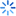• 签到
•苹果/安卓/wp

•苹果/安卓/wp

客户端
STATA10随机效应模型估计的bug
• 统计与数据分析
• 经济学
• 管理学
• 金融学
• 财会类
• 国际贸易类
• 考研考博与考证
• 找数据和资料
• 求职与职场
• 学术与投稿类
• 社会生活
• 其它

STATA10随机效应模型估计的bug0443424
STATA10随机效应模型估计的bug

Random-effects GLS regression                   Number of obs      =        90
Group variable: i                               Number of groups   =         6
R-sq:  within  = 0.9925                         Obs per group: min =        15
between = 0.9856                                        avg =      15.0
overall = 0.9876                                        max =        15
Random effects u_i ~ Gaussian                   Wald chi2(3)       =  11091.33
corr(u_i, X)       = 0 (assumed)                Prob > chi2        =    0.0000
theta              = .87668505
------------------------------------------------------------------------------
lnc |      Coef.   Std. Err.      z    P>|z|     [95% Conf. Interval]
-------------+----------------------------------------------------------------
lnq |   .9066805    .025625    35.38   0.000     .8564565    .9569045
lnpf |   .4227785   .0140248    30.15   0.000     .3952904    .4502665
lf |  -1.064498   .2000703    -5.32   0.000    -1.456629   -.6723678
_cons |   9.627909    .210164    45.81   0.000     9.215995    10.03982
-------------+----------------------------------------------------------------
sigma_u |  .12488861
sigma_e |  .06010514
rho |  .81193821   (fraction of variance due to u_i)
------------------------------------------------------------------------------

1、sigma_u=0.12488861，（sigma_u）^2=0.015597。结果和格林的结果一致。但是在5ed中，格林采用了另外一种方法计算了（sigma_u）^2=0.0119155，然后用这个来计算theta。
2、sigma_e =06010514，这个结果和格林结果（4ed and 5ed）都一致。因为这个就是LSDV中的结果。
3、theta  = 0.87668505。这个结果和格林4ed一致，但是显然格林4ed的计算是错误的，因为page574的第三个计算公式没有按照page569的theta计算公式进行，page574的计算中分母少了一项，这点格林在5ed的勘误中间提到了。正确的计算如下：
1-(0.00361262/(0.00361262+15*(0.0119154)))^0.5=0.85924434加载中...